1. Home
2. Docs
3. Numerical Methods
4. Solution of Nonlinear Equations
5. Newton-Raphson Method

# Newton-Raphson Method

## Introduction

Consider a graph below of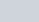. Let us consider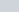is an approximate root of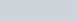. Draw a tangent at the curveat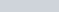as shown in figure aside. The point of intersection of this tangent with the x-axis gives the second approximation to the root. Let the point of intersection be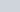. The slope of the tangent is given by;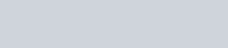where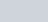is the slope ofat. Solving forwe obtain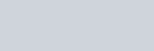This is called the Newton-Raphson formula.
The next approximation would beSo, we can generalize the next approximation using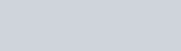And finally the process is repeated until the difference between the consecutive values are within prescribed limit.

## Algorithm

1. Guess initial root =and define stopping criteria error
2. Evaluateand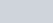3. Compute the new root4. Set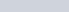5. Check if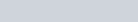goto step 3, else
6. Print root =7. End

## Code

### C

#include <stdio.h>
#include <stdlib.h>
#include <math.h>
#define f(x) ((x * x * x) - 18)
#define fd(x) (3 * x * x)
#define fdd(x) 6 * x
int main()
{
float x0, x1, error, errorold, converge, order;
int i = 0;
printf("Input the approximation : ");
scanf("%f", &x0);
converge = (f(x0) * fdd(x0)) / (fd(x0) * fd(x0));
if (converge > 1)
exit(1);
printf("Ite\tX0\t\tX1\t\tError\t\tOrder\n");
do
{
errorold = error;
x1 = x0 - (f(x0) / fd(x0));
if (f(x1) == 0)
{
break;
}
error = fabs(x1 - x0);
printf("%2d\t%4.6f\t%4.6f\t%4.6f\t", ++i, x0, x1, error);
if (i == 1 || error == 0 || errorold == 1)
{
printf("-----\n");
}
else
{
order = log(error) / log(errorold);
printf("%4.6f\n", order);
}
x0 = x1;
} while (error > 0.00005);
printf("Root is %4.6f", x0);
return 0;
}